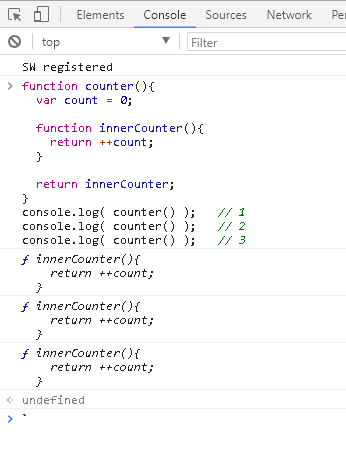#2018 iT 邦幫忙鐵人賽DAY 19
10
Modern Web

## 範圍鏈 Scope Chain

``````function outer() {

// 在 outer 這層拿不到變數 c
// 但可以向外找到變數 a
var b = a * 2;

function inner(c) {
// 由於範圍鍊的關係，雖然只有對 c 定義，
// 但可以像上一層一層取得 a, b, c
console.log(a, b, c);
}

inner(b * 3);
}

// 在 global 這層只有 a, 不認得 b 與 c
var a = 1;
outer(a);
``````

``````function outer() {
// 把 var 拿掉
b = a * 2;

function inner(c) {
console.log(a, b, c);
}

inner(b * 3);
}

var a = 1;
outer(a);
``````

.
.
.

(忘記的同學趕快再回去看: 重新認識 JavaScript: Day 10 函式 Functions 的基本概念 )

``````var msg = "global."

function outer() {
var msg = "local."

function inner() {
return msg;
}

return inner;
}

var innerFunc = outer();
var result = innerFunc();

console.log( result );    //  ?
``````

.
.
.## 閉包 Closure

``````for( var i = 0; i < 5; i++ ) {
(function(i){
window.setTimeout(function() {
console.log(i);
}, 1000 * i);
})(i);
}
``````

``````function outer() {
var msg = "local."

function inner() {
return msg;
}

return inner;
}
``````

``````var count = 0;

function counter(){
return ++count;
}

console.log( counter() );   // 1
console.log( counter() );   // 2
console.log( counter() );   // 3
``````

``````function counter(){
var count = 0;

function innerCounter(){
return ++count;
}

return innerCounter;
}

var countFunc = counter();

console.log( countFunc() );   // 1
console.log( countFunc() );   // 2
console.log( countFunc() );   // 3
``````

``````function counter(){
var count = 0;

return function(){
return ++count;
}
}
``````

``````var counter = () => {
var count = 0;
return () => ++count;
}
``````

``````
function counter(){
var count = 0;

return function(){
return ++count;
}
}

var countFunc = counter();
var countFunc2 = counter();

console.log( countFunc() );   // 1
console.log( countFunc() );   // 2
console.log( countFunc() );   // 3

console.log( countFunc2() );   // 1
console.log( countFunc2() );   // 2
``````

### 2 則留言

1``````function counter(){
var count = 0;

function innerCounter(){
return ++count;
}

return innerCounter;
}
``````

``````var countFunc = counter();
``````

``````function counter(){
var count = 0;

function innerCounter(){
return ++count;
}

return innerCounter;
}

var countFunc = counter();

console.log( countFunc() );   // 1
console.log( countFunc() );   // 2
console.log( countFunc() );   // 3
``````
Luke iT邦新手 3 級‧ 2017-12-28 14:38:30 檢舉
``````function counter(){
var count = 0;

function innerCounter(){
return ++count;
}

var tmpV = innerCounter();
console.log( '>>>' + tmpV );
return tmpV;
}
console.log( counter() );   // 1
console.log( counter() );   // 2
console.log( counter() );   // 3
``````

``````var count = 0;
``````

``````var countFunc = counter();
``````

0

`var contFunc = counter();`
`console.log`去看`contFunc()`執行後的結果，為何會自動回傳數值，而不會是innerCounter()這個function呢?

mingyang

``````function counter() {
var count = 0;

function innerCounter() {
return ++count;
}

return innerCounter;
}

var countFunc = counter();

console.log( countFunc() );   // 1
``````

`countFunc` 這個變數存進來的東西其實是 `counter` 這個 function 回傳的結果，也就是 `innerCounter` 對吧？